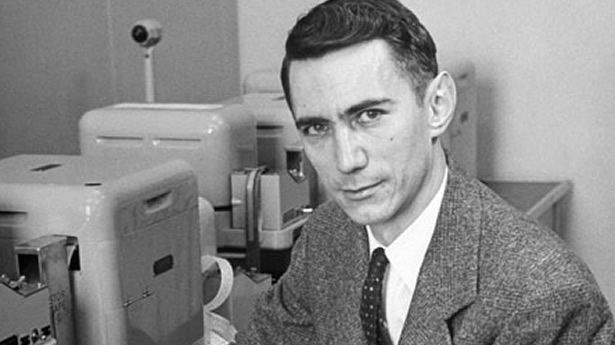This equation was published in the 1949 book The Mathematical Theory of Communication, co-written by Claude Shannon and Warren Weaver. An elegant way to work out how efficient a code could be, it turned "information" from a vague word related to how much someone knew about something into a precise mathematical unit that could be measured, manipulated and transmitted. It was the start of the science of "information theory", a set of ideas that has allowed us to build the internet, digital computers and telecommunications systems. When anyone talks about the information revolution of the last few decades, it is Shannon's idea of information that they are talking about.

Claude Shannon was a mathematician and electronic engineer working at Bell Labs in the US in the middle of the 20th century. His workplace was the celebrated research and development arm of the Bell Telephone Company, the US's main provider of telephone services until the 1980s when it was broken up because of its monopolistic position. During the second world war, Shannon worked on codes and methods of sending messages efficiently and securely over long distances, ideas that became the seeds for his information theory.

Before information theory, remote communication was done using analogue signals. Sending a message involved turning it into varying pulses of voltage along a wire, which could be measured at the other end and interpreted back into words. This is generally fine for short distances but, if you want to send something across an ocean, it becomes unusable. Every metre that an analogue electrical signal travels along a wire, it gets weaker and suffers more from random fluctuations, known as noise, in the materials around it. You could boost the signal at the outset, of course, but this will have the unwanted effect of also boosting the noise.

Information theory helped to get over this problem. In it, Shannon defined the units of information, the smallest possible chunks that cannot be divided any further, into what he called "bits" (short for binary digit), strings of which can be used to encode any message. The most widely used digital code in modern electronics is based around bits that can each have only one of two values: 0 or 1.

This simple idea immediately improves the quality of communications. Convert your message, letter by letter, into a code made from 0s and 1s, then send this long string of digits down a wire – every 0 represented by a brief low-voltage signal and every 1 represented by a brief burst of high voltage. These signals will, of course, suffer from the same problems as an analogue signal, namely weakening and noise. But the digital signal has an advantage: the 0s and 1s are such obviously different states that, even after deterioration, their original state can be reconstructed far down the wire. An additional way to keep the digital message clean is to read it, using electronic devices, at intervals along its route and resend a clean repeat.

Shannon showed the true power of these bits, however, by putting them into a mathematical framework. His equation defines a quantity, H, which is known as Shannon entropy and can be thought of as a measure of the information in a message, measured in bits.

In a message, the probability of a particular symbol (represented by "x") turning up is denoted by p(x). The right hand side of the equation above sums up the probabilities of the full range of symbols that might turn up in a message, weighted by the number of bits needed to represent that value of x, a term given by logp(x). (A logarithm is the reverse process of raising something to a power – we say that the logarithm of 1000 to base 10 – written log10(1000) – is 3, because 103=1000.)

A coin toss, for example, has two possible outcomes (or symbols) – x could be heads or tails. Each outcome has a 50% probability of occurring and, in this instance, p(heads) and p(tails) are each ½. Shannon's theory uses base 2 for its logarithms and log2(½) is -1. That gives us a total information content in flipping a coin, a value for H, of 1 bit. Once a coin toss has been completed, we have gained one bit of information or, rather, reduced our uncertainty by one bit.

A single character taken from an alphabet of 27 has around 4.76 bits of information – in other words log2(1/27) – because each character either is or is not a particular letter of that alphabet. Because there are 27 of these binary possibilities, the probability of each is 1/27. This is a basic description of a basic English alphabet (26 characters and a space), if each character was equally likely to turn up in a message. By this calculation, messages in English need bandwidth for storage or transmission equal to the number of characters multiplied by 4.76.

But we know that, in English, each character does not appear equally. A "u" usually follows a "q" and "e" is more common than "z". Take these statistical details into account and it is possible to reduce the H value for English characters to less than one bit. Which is useful if you want to speed up comms or take up less space on a hard disk.

Information theory was created to find practical ways to make better, more efficient codes and find the limits on how fast computers could process digital signals. Every piece of digital information is the result of codes that have been examined and improved using Shannon's equation. It has provided the mathematical underpinning for increased data storage and compression – Zip files, MP3s and JPGs could not exist without it. And none of those high-definition videos online would have been possible without Shannon's mathematics.

["Claude Shannon" via Wikimedia Commons]